# Lab Introduction - ADC DAC Schematic and simulation

In this guided laboratory, our task is to make some simulation and analysis of a ramp and sine signal applied to an electronic circuit that consists of both ADC and DAC circuits inside, which is designed in LTspice. Our basic expectation is to get an output that is similar to the input, and then we will see how much deviation we have by using error analysis techniques like DNL, INL, LSB for the ramp and FFT, DNL, INL, SNR for the sine signal. These will give us clear information for the practical implementation of the ADC DAC circuit, and we will see whether the behavior is the same or not.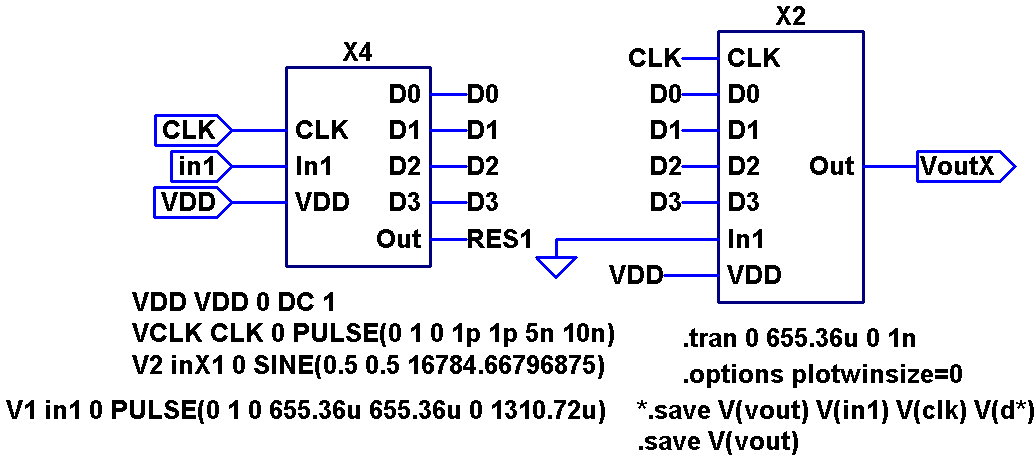The ADC-DAC circuit is shown in the above figure. So by using the voltage V1 or V2, we can do the ramp and sine signal test in LTspice. The result of this simulation is shown below. In both inputs, the input signal is a smooth analog signal and the output is a kind of staircase because of the discretization. We have also an FFT simulation option in LTspice and from that, we were abe to see the signal to noise ratio of the input signal but we couldn't see the SNR of the output due to the case that LTspice doesn’t provide us with all the values for our data converter analysis.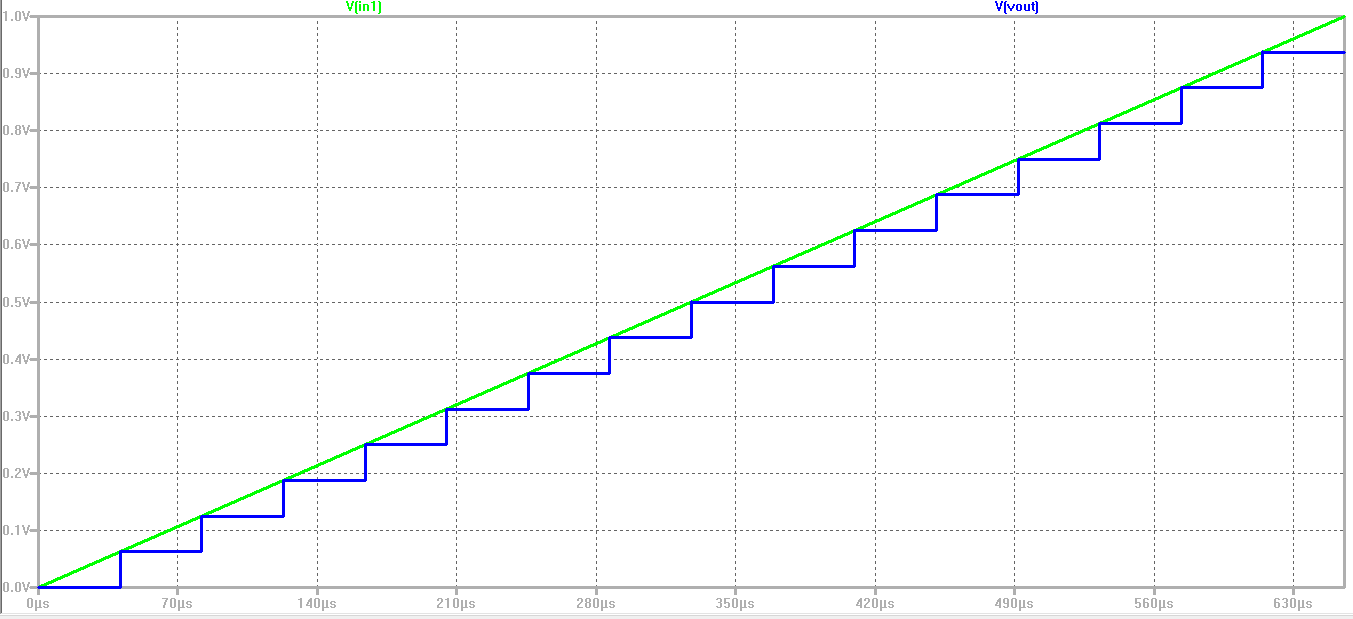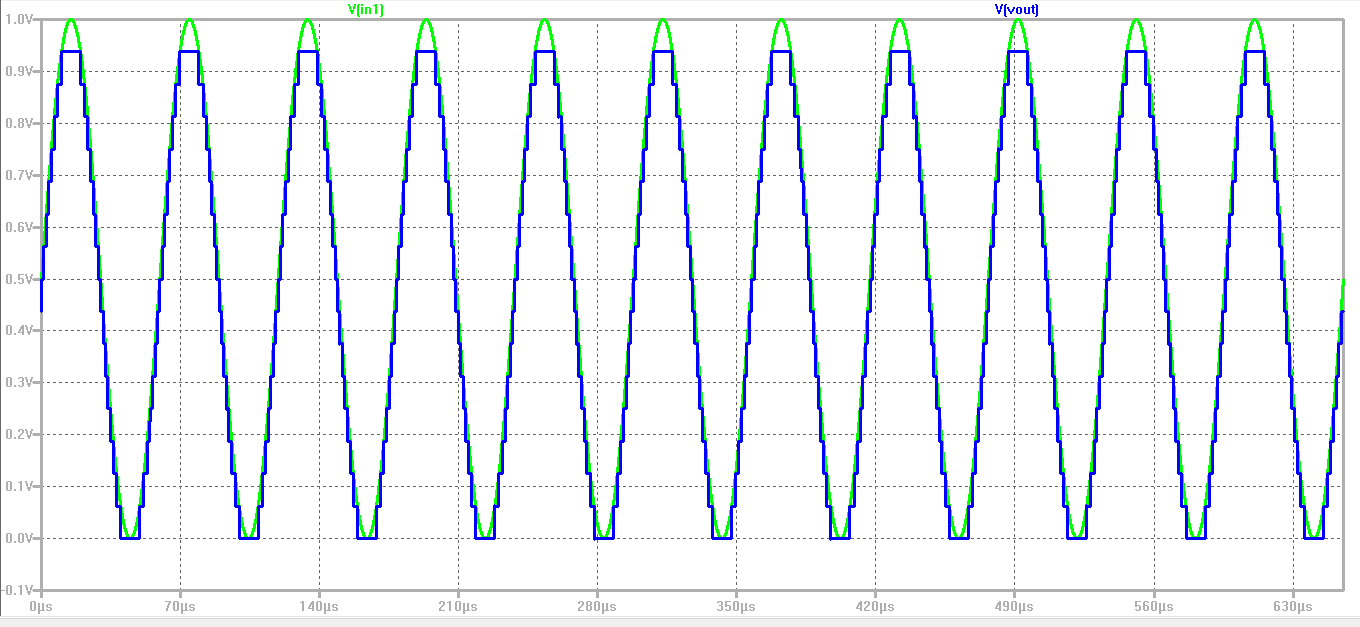FFT of the input and output of the sine signal from LTspice.# Ramp Signal Test

Since we have a long step size in LTspice and we need to take samples from each digital level, so instead of going for .meas operation, it will be easier to extract and filter the data by the javascript tool provided in the interface webpage. So for the first simulation of the ramp signal, the extracted files are aligned with the ideal values (these values are from the time step calculated by total simulation time divided by the number of steps). Therefore all the INL and DNL of the DAC test are zero as shown below.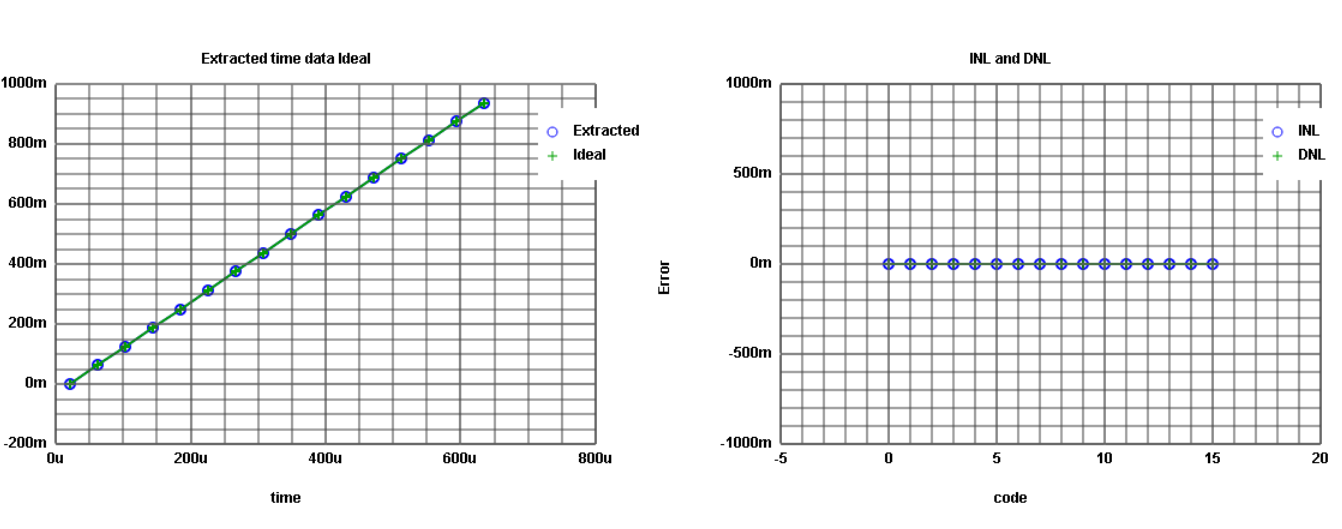Then we do a histogram test for ADC analysis. For the histogram test, we increase the number of steps so that we can see how many times each digital code word appears on the ADC output. Since we are still in the ideal case the result of the histogram test is with zero DNL and INL shown below.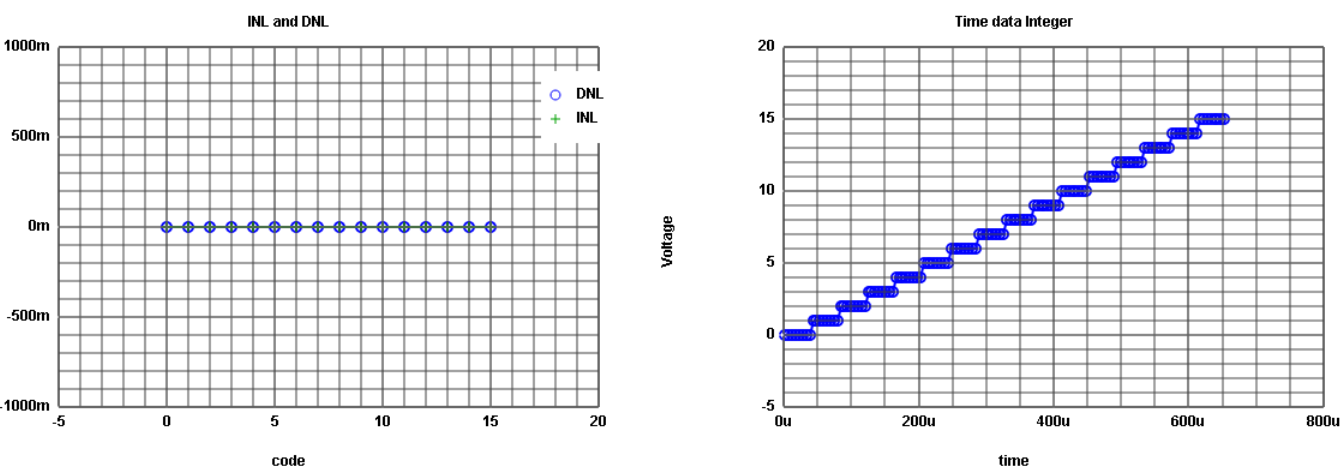# Sine Signal Test

 First, we get the extracted values and now we can do INL and DNL test. But the data are from constant average values, not real values. So for better analysis, we do the FFT analysis and then we saw the signal to noise ratio. The signal frequency was 11 Hz and from the result, we got the signal magnitude 11.87dB and total noise magnitude -13.89dB. Since our SNR is 25.76 then our effective number of bits will be 25.76 / 6.02 = 4Bits the same as what we have implemented.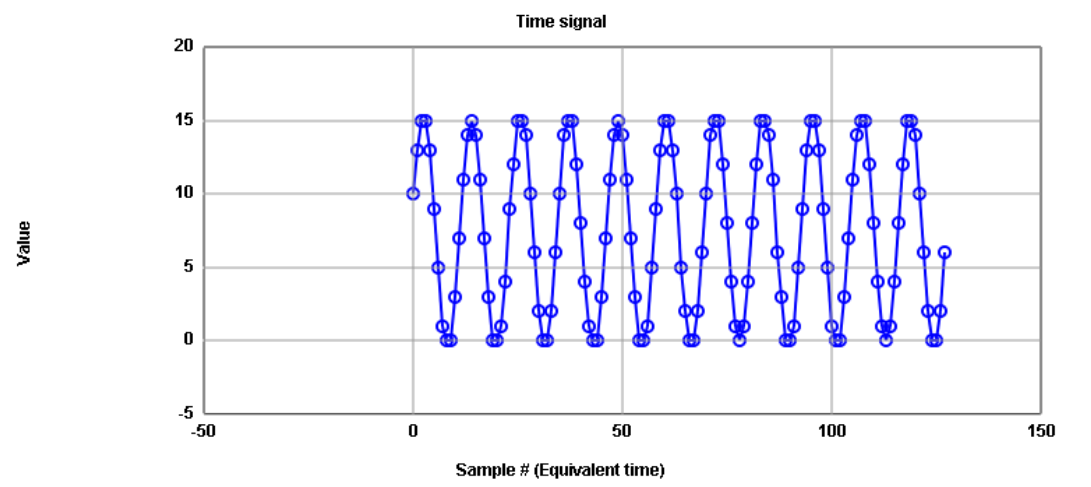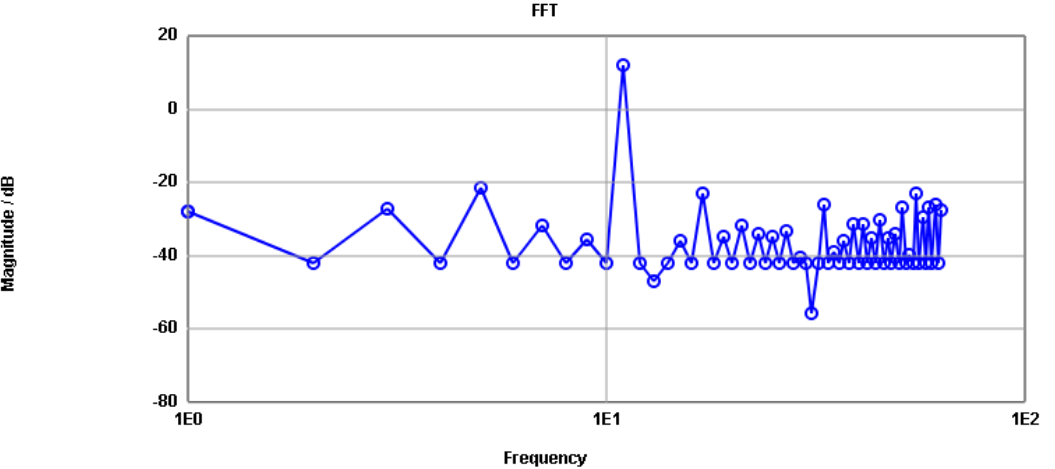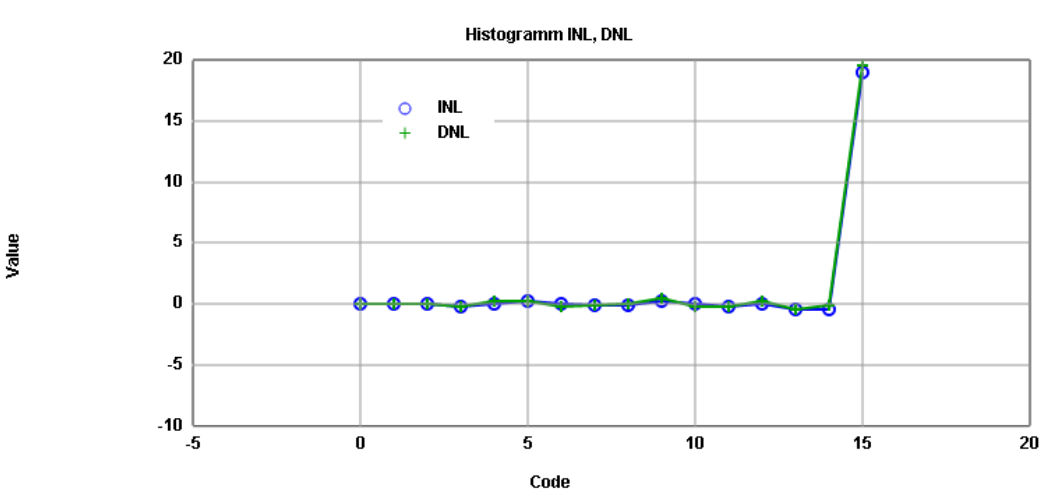In the above result, I was expecting the final value of the INL to be at zero and we have discussed that there could be some errors. But later on, we saw how different offset and amplitude of our input signal can influence the DNL and INL results. So we need to consider that we have to make the best fit for our sine signal to get the correct result. After the JavaScript corrected, the result is shown below: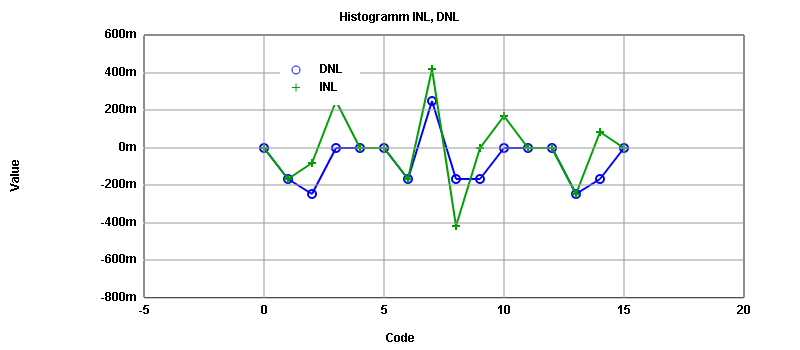# Simulation R2R DAC

Now we have replaced our DAC converter to R2R circuit and after simulation the ideal output is as shown below.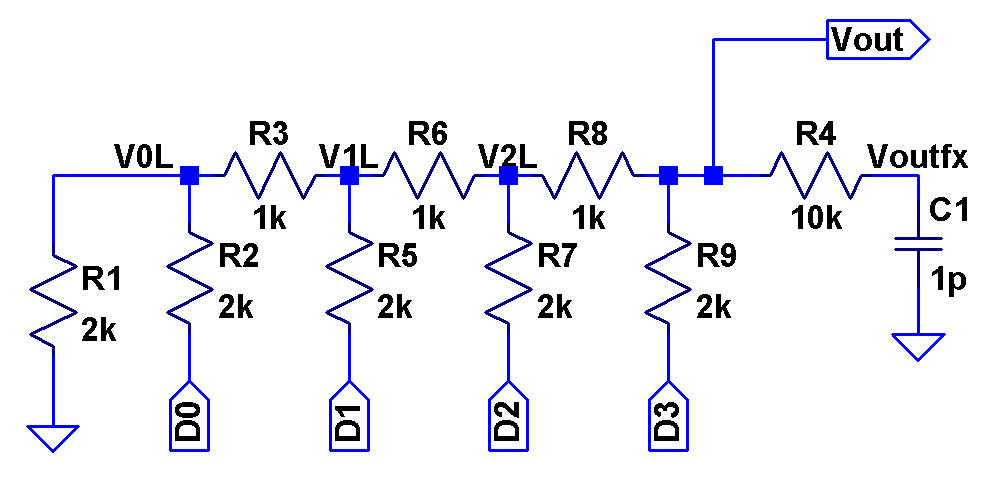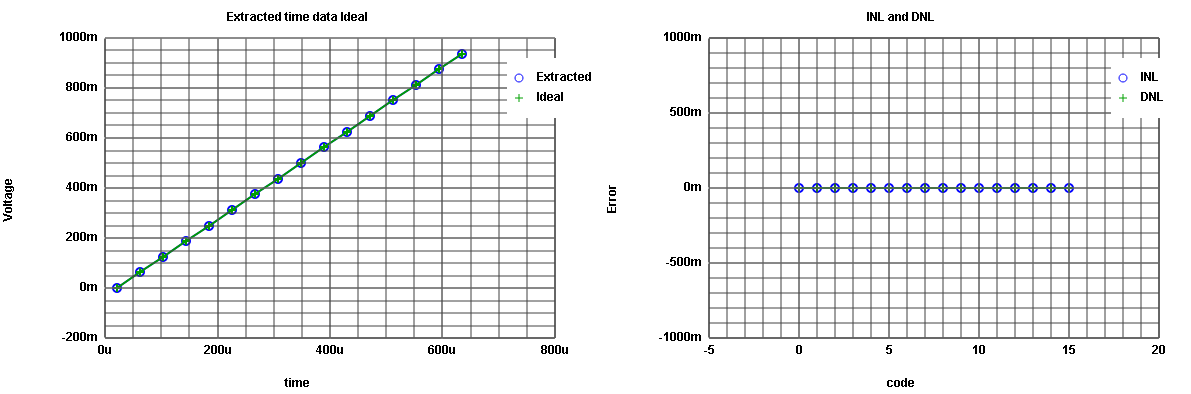For further investigation, we have changed the resistance R6 to 1.3k and R9 to 1.5k. Then from the figure below we can see how much our output curve and DNL, INL are changed. In order to see the effect, we made a big change in the resistance. The change in the resistance could be due to temperature. In reality, there is no significant change. But even though the change is small it can be easily detected by the INL DNL simulation. The result of this new resistance value is shown here. We can see the big jump in the middle (due to the highest order bit resistance change’ R9’) and the two terms of the lower order bit on the right and left (due to R6).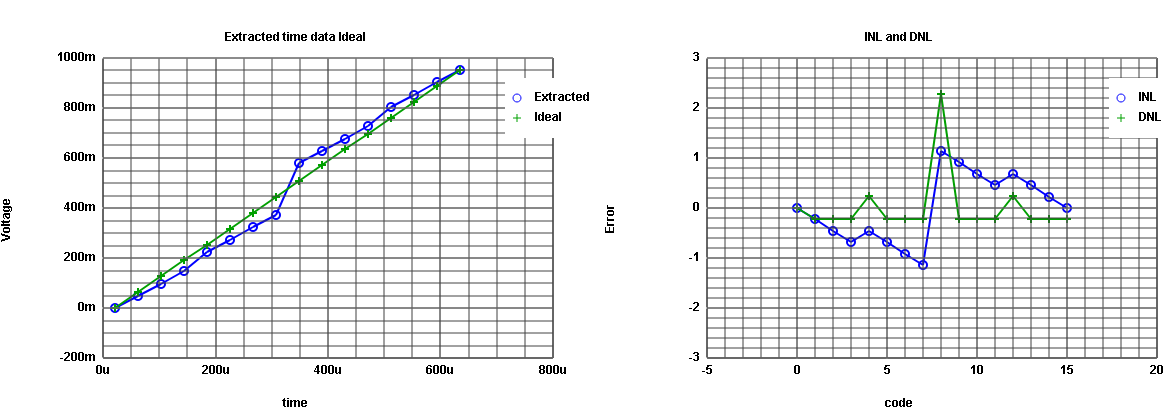# Learning Outcomes and Challenges

From this lab, I understand more details on how to convert an analog signal to a digital signal and vice versa. I learn how we can take samples and what possible errors can occur. Then by applying a ramp and sine signals, I have learned how I can measure the performance of ADC DAC converter with DNL, INL, SNR (FFT). Then for real circuit simulation, we consider the R2R DAC circuit, and we also assume that in reality the resistance value can be changed and this change causes errors but then we successfully detect these errors by the DNL INL test and this can be debugged by adjusting the values of the resistors.

Moreover, I have also upgraded my knowledge of Webpage editing.

Time Spent

I spent about twelve hours to complete this laboratory. Eight hours to simulate and understand the concept, 2 hours to write and edit it, and last two hours to put it on a webpage.

# References

 Laboratory guide 03 , Interface Electronics website, Hochschule Kempten , Prof.Vollrath

 Making of a Webreport , Prof.Vollrath

 Lecture classes, Prof. Vollrath